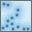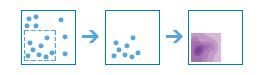Calculate DensityThe Calculate Density tool uses input point or line features to calculate a density map within an area of interest.

Workflow diagramExamples

• Bird counts can be used to calculate species densities. The densities can then be compared to land-cover data to determine which habitats each species prefers.
• The density of flight paths can be calculated to determine which areas of air space have high volumes of traffic.

Usage notes

The Calculate Density tool requires a single input of point or line features.

Density can optionally be calculated using a count field. A count field is a numerical field that specifies the number of incidents at each location. Features such as cities or highways could use a count field when calculating the density of population or lanes of traffic, respectively.

By default, the Calculate Density tool will calculate an appropriate search distance for determining neighborhood size, will not clip the output, will classify the output as Equal Interval with 10 classes, and will provide the output densities in Square Miles or Square Kilometers, depending on the Units setting in your profile. These defaults can be changed using the Options drop-down menu.

OptionDescriptionDefault

Search Distance

A distance (in miles, feet, kilometers, or meters) that is used to find input features within the same neighborhood as the focal feature.

An appropriate search distance will be calculated using the locations of the input features.

Clip output to

An area from a layer or the draw tool can be used to specify an area of interest. The density will be calculated and then clipped to the boundaries of the area.

None

Classify by

The classification scheme used to display the resulting density layer. Options include Equal Interval, Equal Area, Geometric Interval, Natural Breaks, and Standard Deviation.

Equal Interval

Number of classes

The number of classes to be used in the result layer. Used with the classification scheme in the Classify by option.

10

Output area units

The units of the area used to calculate density. Options include Square Miles and Square Kilometers.

Square Miles (US Standard setting) or Square Kilometers (Metric setting).

Limitations

Density can be calculated for point and line features only.

Similar tools

Use Calculate Density to create a density map using point or line measurements. Other tools may be useful in solving similar but slightly different problems.

Map viewer analysis tools

If you want to find statistically significant clustering in point or area features, use the Find Hot Spots tool.

If you want to predict values based on point measurements, use the Interpolate Points tool.

ArcGIS Desktop analysis tools

The Calculate Density tool performs a similar function to the Kernel Density, Line Density, and Point Density tools.

Calculate Density is also available in ArcGIS Pro. To run the tool from ArcGIS Pro, your project's active portal must be running Portal for ArcGIS 10.5 or later. You must also sign in to the portal using an account that has privileges to perform standard feature analysis in the portal.